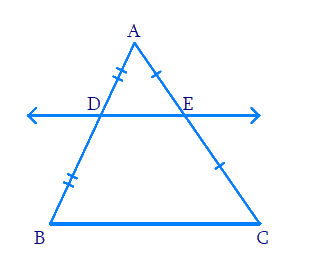# Ex.6.2 Q8 Triangles Solution - NCERT Maths Class 10

Go back to  'Ex.6.2'

## Question

Using Theorem $$6.2$$, prove that the line joining the mid-points of any two sides of a triangle is parallel to the third side. (Recall that you have done it in Class IX).Video Solution
Triangles
Ex 6.2 | Question 8

## Text Solution

Reasoning:

We know that theorem $$6.2$$ tells us if a line divides any two sides of a triangle in the same ratio, then the line is parallel to the third side. (Converse of $$BPT$$)

Steps:

In $$\Delta ABC$$

$$D$$ is the midpoint of $$AB$$

\begin{align}AD &= BD\\ \frac{AD}{BD} &= 1\;\dots\rm{(i)}\end{align}

$$E$$ is the midpoint of $$AC$$

\begin{align} AE &= CE\\ \frac{AE}{BE} &= 1\;\dots\rm{(ii)}\end{align}

From (i) and (ii)

\begin{align} \frac{AD}{BD}&=\frac{AE}{BE} = 1 \\ \frac{AD}{BD}&=\frac{AE}{BE} \\\end{align}

According to theorem $$6.2,$$ (Converse of $$BPT$$)

$DE||BC$

Learn from the best math teachers and top your exams

• Live one on one classroom and doubt clearing
• Practice worksheets in and after class for conceptual clarity
• Personalized curriculum to keep up with school